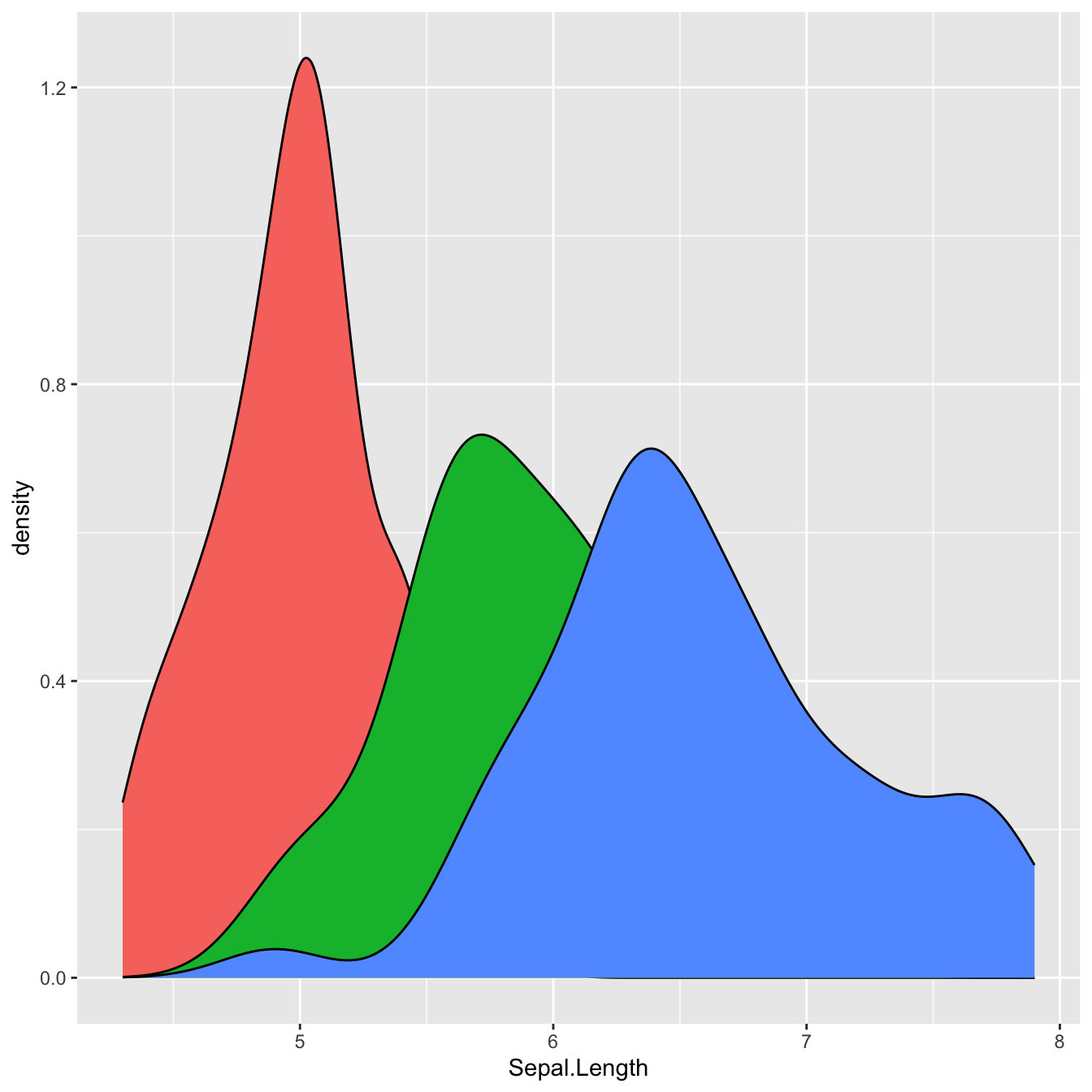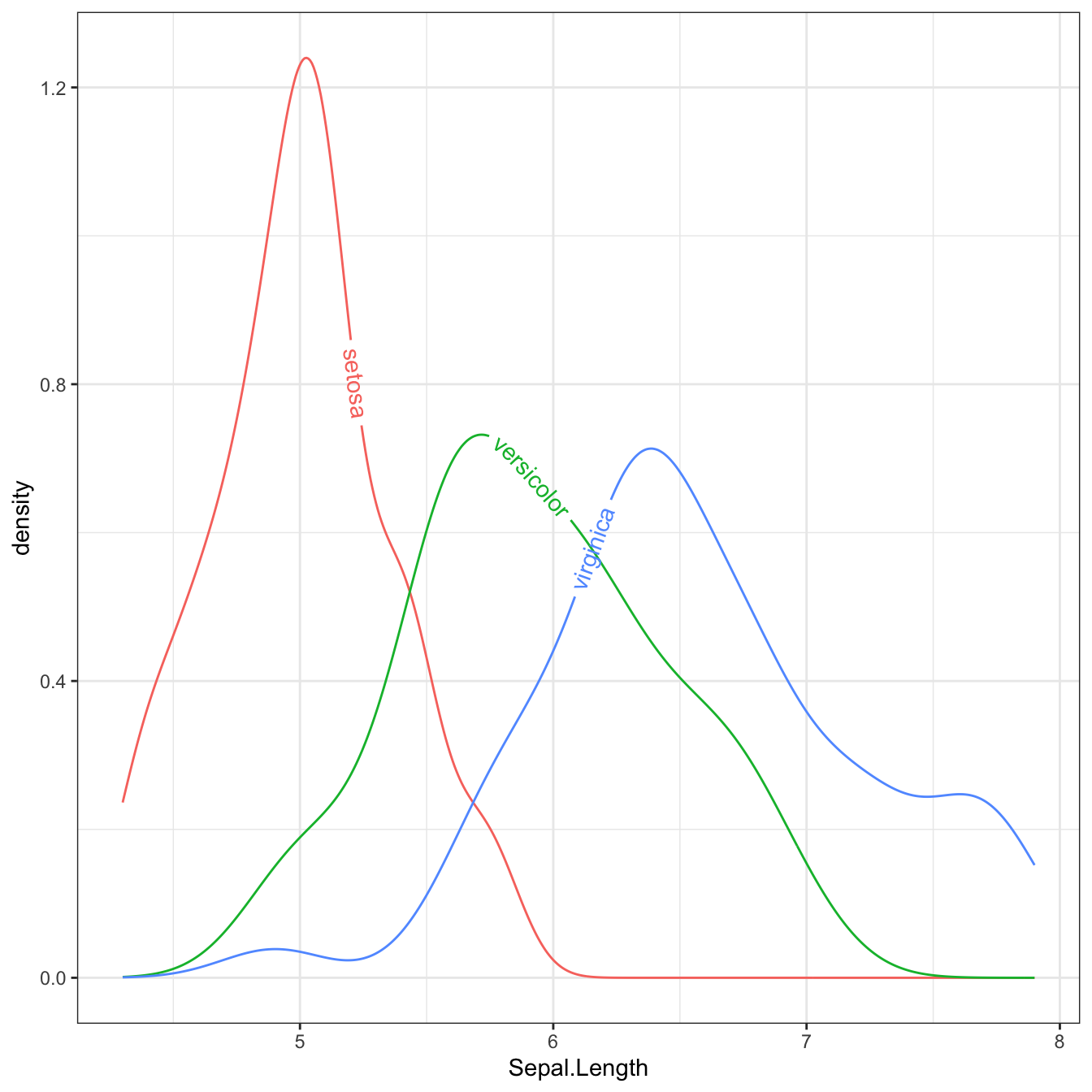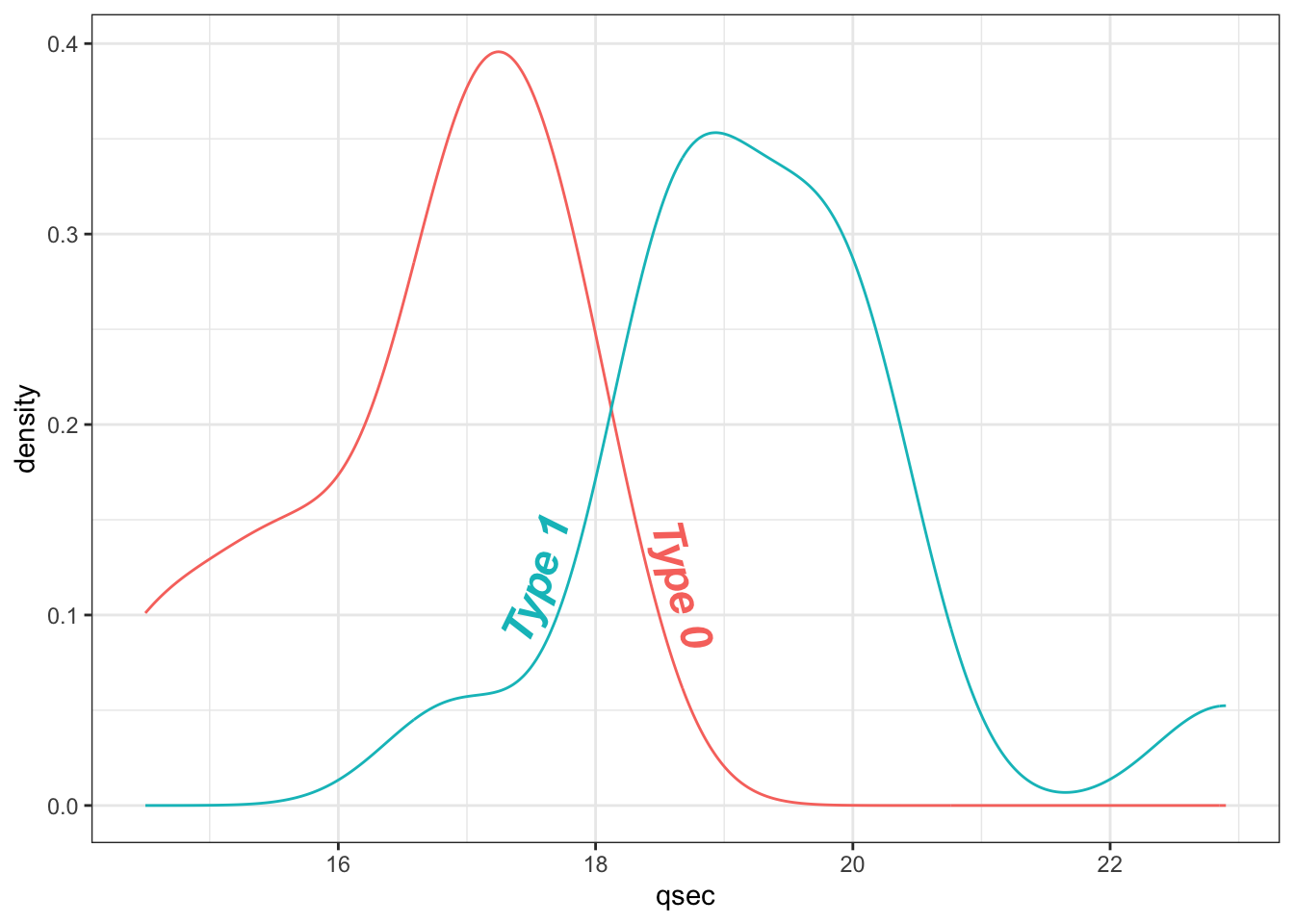# Density plot with labels on lines

This post explains how to add a text or labels on the line of a density chart. It uses the ggplot2 and geomtextpath packages for creating the chart and making it nice.

# Package

For this post, we need the ggplot2 and geomtextpath packages.

``````# Install the packages if not already done
# install.packages("ggplot2")
# install.packages("geomtextpath")

library(ggplot2)
library(geomtextpath)``````

# Default density chart in ggplot2

Here’s what the default density chart output looks like with `ggplot2`:With the `geom_textdensity()` function (inspired from the `geom_density()` func from ggplot2), we can add the label of species directly on each density line.

``````library(hrbrthemes)
data(iris)

ggplot(iris, aes(x = Sepal.Length, colour = Species, label = Species)) +
geom_textdensity() +
theme_bw() + guides(color = 'none')``````# Change texts properties

The `geom_textdensity()` can take multiple arguments in order to change the properties of the labels:

• `size`: size of the text

• `fontface`: style of the font

• `vjust`: vertical adjustment

• `hjust`: horizontal adjustment

The 2 last arguments can either be float (generally between -1 and 1) or string such as `xmid` (or `ymid`), `xmax` (or `ymax`) and `auto` (default).

``````library(hrbrthemes)
data(mtcars)

mtcars\$labels = ifelse(mtcars\$vs==0, "Type 0", "Type 1")
ggplot(mtcars, aes(x = qsec, colour = as.factor(labels), label = as.factor(labels))) +
geom_textdensity(size = 6, fontface = 4, # size of 6, bold italic
vjust = -0.4, hjust = "ymid") +
theme_bw() + guides(color = 'none')``````# Conclusion

In this post, we look at how to use the geomtexpath package to create density plot with text. To find out more about how to customize a density plot, see the dedicated section.

Related chart types

## Contact

This document is a work by Yan Holtz. Any feedback is highly encouraged. You can fill an issue on Github, drop me a message on Twitter, or send an email pasting yan.holtz.data with gmail.com.